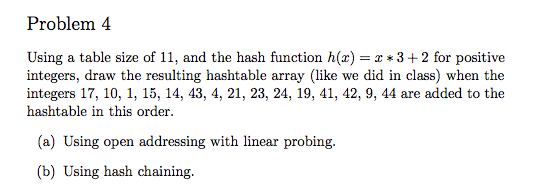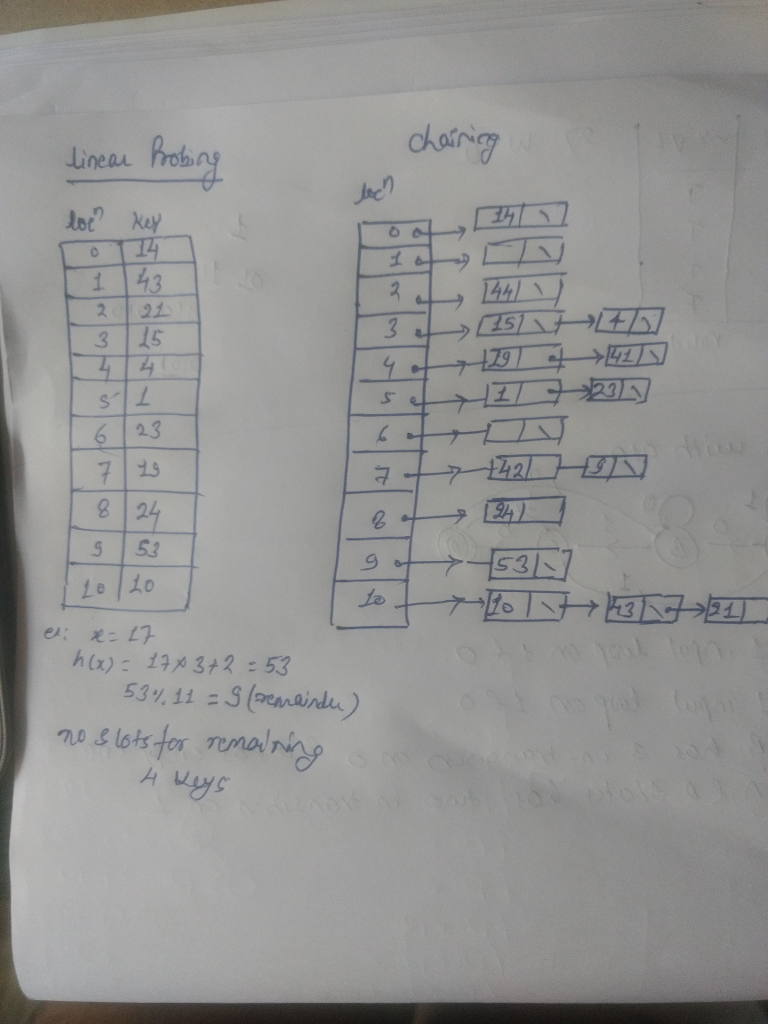# Answered! Using a table size of 11, and the hash function h{x) = x * 3 + 2 for positive integers, draw the resulting hashtable array…Using a table size of 11, and the hash function h{x) = x * 3 + 2 for positive integers, draw the resulting hashtable array (like we did in class) when the integers 17, 10, 1, 15, 14, 43, 4, 21, 23, 24, 19, 41, 42. 9, 44 are added to the hashtable in this order. (a) Using open addressing with linear probing. (b) Using hash chaining.